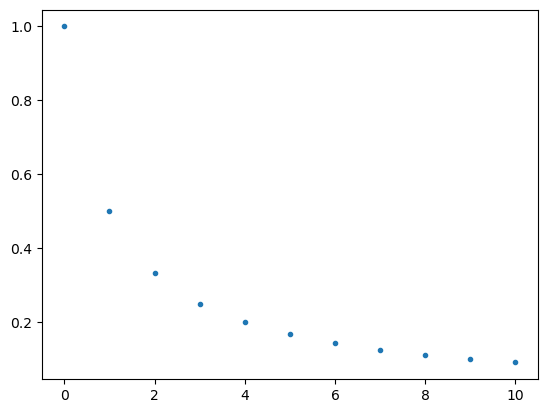# How to graph mathematical sequences

## Description

Assume we have a mathematical sequence $a_0,a_1,a_2,\ldots$ and we would like to plot a graph of it using the standard Cartesian coordinate system. The result will not look like a curve, because a sequence is separate points instead of a smooth curve.

## Using SymPy and Matplotlib, in Python

View this solution alone.

This answer assumes you have imported SymPy as follows.

1
2
from sympy import *                   # load all math functions
init_printing( use_latex='mathjax' )  # use pretty math output


We will re-use the sequence defined in the task how to define a mathematical sequence.

1
2
3
4
var( 'n' )
a_n = 1 / ( n + 1 )
seq = sequence( a_n, (n,0,oo) )
seq


$\displaystyle \left[1, \frac{1}{2}, \frac{1}{3}, \frac{1}{4}, \ldots\right]$

We can graph any finite range of any sequence as follows.

1
2
3
4
5
start = 0
stop = 10
import matplotlib.pyplot as plt
plt.plot( range(start,stop+1), seq[start:stop+1], '.' )
plt.show()See a problem? Tell us or edit the source.

## Opportunities

This website does not yet contain a solution for this task in any of the following software packages.

• R
• Excel
• Julia

If you can contribute a solution using any of these pieces of software, see our Contributing page for how to help extend this website.